# Plus Two Chemistry Solution Chapter1 The Solid State

keralanotes.com com has been providing a complete solution for all the chapters and subjects of the Kerala syllabus plus two chemistry students. now we are even more advanced and have introduced the most detailed chapter-wise textbook solutions  This is published and updated by our expert team of teachers who are working at reputed schools for decades.

keralanotes.com provides the latest and detailed textbook solutions for the Kerala syllabus plus two chemistry students. It's sorted in a way that all answers are easily understandable, searchable, and printable. It contains questions with page numbers and marks for easy searching.

 Board SCERT, Kerala Text Book NCERT Based Class Plus Two Subject Chemistry Textbook Solution Chapter Chapter 1 Chapter Name The Solid State Category Plus Two Kerala

## Kerala Syllabus Plus Two Chemistry Textbook Solution Chapter  1 The Solid State

### Plus Two Chemistry Chapter 1 The Solid State INTEXT Questions

Question 1.
Why are solids rigid?
Solution:
Unlike gases and liquids in which the molecules are free to move about and hence constitute a fluid state, in a solid, the constituent particles are not free to move but oscillate about their fixed positions providing them a rigid structure.

Question 2.
Why do solids have a definite volume?
Solution:
The constituent particles in solids are bound to their mean positions by strong cohesive forces of attraction. The interparticle distances remain unchanged at a given temperature and thus solids have a definite volume.

Question 3.
Classify the following as amorphous or crystalline solids: Polyurethane, naphthalene, benzoic acid, Teflon, potassium nitrate, cellophane, polyvinyl chloride, fiberglass, copper.
Solution:
Amorphous solids: Polyurethane, Teflon, cellophane, polyvinyl chloride and fiber glass
Crystalline solids: Naphthalene, benzoic acid, potassium nitrate, and copper

Question 4.
Why is glass considered a supercooled liquid?
Solution:
Glass is an amorphous solid. Like liquids, it has a tendency to flow, though very slowly. This is evident from the fact that the glass panes in the windows or doors of old buildings are invariably found to be slightly thicker at the bottom than at the top. This is because the glass flows down very slowly and makes the bottom portion slightly thicker.

Question 5.
The Refractive index of a solid is observed to have the same value along with all directions. Comment on the nature of this solid. Would it show cleavage property?
Solution:
Since the solid has the same value of refractive index along with all directions, it is isotropic in nature. It is because there is no long-range order and the arrangement is irregular along with all the directions and hence, amorphous. Being an amorphous solid, it would not show a clean cleavage when cut with a knife. Instead, it would break into pieces with irregular surfaces.

Question 6.
Classify the following solids in different categories based on the nature of intermolecular forces operating in them :
potassium sulphate, tin, benzene, urea, ammonia, water, zinc sulphide, graphite, rubidium, argon, silicon carbide.
Solution:
Ionic solids: Potassium sulfate, zinc sulfide
Covalent solids: Graphite, silicon carbide
Molecular solids: Benzene, urea, ammonia, water, argon
Metallic solids: Rubidium, tin

Question 7.
Solid A is a very hard electrical insulator in solid as well as in molten state and melts at extremely high temperature. What type of solid is it?
Solution:
Covalent or network solid

Question 8.
Ionic solids conduct electricity in a molten state but not in a solid-state. Explain.
Solution:
Ionic solids conduct electricity in a molten state since, in the molten state, ionic solids dissociate to give free ions. However, in the solid-state, since the ions are not free to move about but remain held together by strong electrostatic forces of attraction, they do not conduct electricity.

Question 9.
What type of solids are electrical conductors, malleable and ductile?
Solution:
Metallic solids conduct electricity in solid-state and are malleable and ductile.

Question 10.
Give the significance of a ‘lattice point’.
Solution:
Constituent particles of a crystalline solid are arranged in a definite fashion in a three-dimensional crystal lattice. Each position that is occupied by the atoms, ions or molecules in the crystal lattice is called a lattice point.

Question 11.
Name the parameters that characterize a unit cell.
Solution:
The size and shape of a unit cell are determined by the lengths of the edges of the unit cell (a, b, and c) which may or may not be mutually perpendicular and by the angles α, β, and y between the edges b and c, c and a and a and b respectively.

Question 12.
Distinguish between
(i) Hexagonal and monoclinic unit cells
(ii) Face-centred and end-centered unit cells.
Solution:
(i) (a) Hexagonal unit cell: a = bc
α = β = 90°, y = 120°
Examples are graphite, ZnO.

(b) Monoclinic unit cell: a ≠ b ≠ c
α = y = 90°, β ≠ 90°
Examples are Na2SO4.10H2O, monoclinic S.

(ii) (a) Face-centred unit cell: Lattice points are at the corners and center of each face.
No. of atoms per unit cell = 8 ×$\frac { 1 }{ 8 }$ + 6 ×$\frac { 1 }{ 2 }$ = 4

(b) End-centred unit cell: Lattice points are at the corners and at the centers of two end faces.
No. of atoms per unit cell = 8 ×$\frac { 1 }{ 8 }$ + 2 ×$\frac { 1 }{ 2 }$ = 2

Question 13.
Explain how many portions of an atom located at (i) corner and (ii) body-center of a cubic unit cell is part of its neighboring unit cell.
Solution:

1. A point lying at the corner of a unit cell is shared equally by eight unit cells and therefore, only one-eighth (1/8) of each such point belongs to the given unit cell.
2. A body-centered point belongs entirely to one unit cell since it is not shared by any other unit cell.

Question 14.
What is the two-dimensional coordination number of a molecule in a square close-packed layer?
Solution:
In such an arrangement, each sphere is in contact with four of its neighbors. Thus, the two-dimensional coordination number is four.

Question 15.
A compound forms a hexagonal close-packed structure. What is the total number of voids in 0. 5 mol of it? How many of these are tetrahedral voids?
Solution:
Number of atoms in 0.5 mol close packing = 0.5 × 6.022 × 1023 = 3.011 × 1023
Number of octahedral voids = Number of atoms in the packing = 3.011 × 1023
Number of tetrahedral voids = 2 × Number of atoms in the packing = 2 × 3.011 × 1023 = 6.022 × 1023
Total number of voids = 3.011 × 1023 + 6.022 × 1023 = 9.033 × 1023

Question 16.
A compound is formed by two elements M and N. The element N forms ccp and atoms of M occupy 1 /3rd of tetrahedral voids. What is the formula of the compound?
Solution:
Suppose no. of atoms of element N = a
Number of tetrahedral voids = 2a
Number of atoms of element M =$\frac { 1 }{ 3 }$ × 2a =$\frac { 2a }{ 3 }$
Ratio of M and N =$\frac { 2a }{ 3 }$ ; a = 2 : 3
The formula of the compound = M2N3

Question 17.
Which of the following lattices has the highest packing efficiency (i) simple cubic (ii) body-centered cubic and (iii) hexagonal close-packed lattice?
Solution:

1. Simple cubic – 52%
2. Body-centered cubic – 68%
3. Hexagonal close-packed – 74%

Hence hcp lattice has the highest packing efficiency.

Question 18.
An element with a molar mass 2.7 × 10-2 kg mol-1 forms a cubic unit cell with an edge length of 405 pm. If its density is 2.7 × 103 kg m-3, what is the nature of the cubic unit cell?
Solution: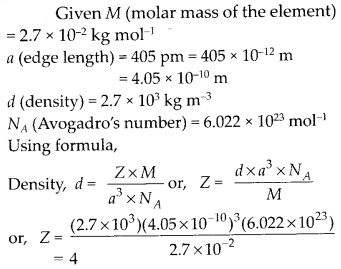The number of atoms of the element present per unit cell = 4. Hence, the cubic unit cell must be face-centered or cubic dose packed (ccp).

Question 19.
What type of defect can arise when a solid is heated? Which physical property is affected by it and in what way?
Solution:
On heating, a solid, vacancy defect is produced in the crystal. This is because, on heating, some lattice sites become vacant. As a result of this defect, the density of the substance decreases because some atoms or ions leave the crystal completely.

Question 20.
What type of stoichiometric defect is shown by:

1. ZnS
2. AgBr

Solution:

1. ZnS – Frenkel defect
2. AgBr – Both Frenkel and Schottky defect

Question 21.
Explain how vacancies are introduced in an ionic solid when a cation of higher valency is added as an impurity in it.
Solution:
When a cation of higher valency is added as an impurity in the ionic solid, some of the sites of the original cations are occupied by the cations of higher valency. Each cation of higher valency replaces two or more original cations and occupies the site of one original cation and the other site(s) remains vacant.

Question 22.
Ionic solids, which have anionic vacancies due to metal excess defect, develop color. Explain with the help of a suitable example.
Solution:
A negative ion may be missing from its lattice site, leaving a hole that is occupied by an electron, thereby maintaining the electrical balance. The electrons thus trapped in the anion vacancies are called E-centres because they are responsible for imparting color to the crystals.For example, when NaCl is heated in an atmosphere of Na vapor, the excess of Na atoms deposit on the surface of the NaCl crystal. The Cl ions diffuse to the surface of the crystal and combine with Na atoms to give NaCl. This happens by the loss of electrons by sodium atoms to form Na+ ions. The released electrons diffuse into the crystal and occupy anionic sites. As a result the crystal now has an excess of sodium. The anionic sites are occupied by unpaired electrons.

They impart a yellow color to the crystals of NaCl. The color results from the excitation of these electrons when they absorb energy from the visible light falling on crystals.Question 23.
A group 14 element is to be converted into an n-type semiconductor by doping it with a suitable impurity. To which group should this impurity belong?
Solution:
n-type semiconductor means conduction due to the presence of excess electrons. Therefore, to convert group 14 element into n-type semiconductor, it should be doped with group 15 element.

Question 24.
What type of substances would make better permanent magnets, ferromagnetic or ferrimagnetic. Justify your answer.
Solution:
Ferromagnetic substances make better permanent magnets. This is because the metal ions of a ferromagnetic substance are grouped into small regions called ‘domains’. Each domain acts as a tiny magnet. These domains are randomly oriented. When a ferromagnetic substance is placed in a magnetic field, all the domains get oriented in the direction of the magnetic field and a strong magnetic field is produced. Such order of domains persists even when the external magnetic field is removed. Hence, the ferromagnetic substance becomes a permanent magnet.

### Plus Two Chemistry Chapter 1 The Solid State  – NCERT Exercises

Question 1.
Define the term ‘amorphous’. Give a few examples of amorphous solids.
Solution:
A solid is said to be amorphous if the constituent particles are not arranged in any regular fashion. They may have only short-range order. Amorphous solids are generally obtained when the melts are rapidly cooled, e.g., glass, plastics, amorphous silica, etc.

Question 2.
What makes a glass different from a solid such as quartz? Under what conditions could quartz be converted into glass?
Solution:
Glass is an amorphous solid in which the constituent particles (Si04 tetrahedra) have only a short-range order and there is no long-range order. In quartz, the constituent particles (SiO4 tetrahedra) have both short-range as well as long-range orders. On melting quartz and then cooling it rapidly, it is converted into glass.

Question 3.
Classify each of the following solids as ionic, metallic, molecular, network (covalent) or amorphous.

1. Tetraphosphorus dioxide, P4O10
2. Ammonium phosphate, (NH4)3PO4
3. SiC
4. l2
5. P4
6. Plastic
7. Graphite
8. Brass
9. Rb
10. LiBr
11. Si

Solution:
P4O10 – molecular, (NH4)3PO4 – ionic, SiC – network (covalent), I2 – molecular, P4 – molecular, plastic – amorphous, graphite – covalent, brass – metallic, Rb – metallic, LiBr – ionic, Si – covalent

Question 4.
(i) What is meant by the term ‘coordination number’?
(ii) What is the coordination number of atoms:
(a) in a cubic close-packed structure?
(b) in a body-centered cubic structure?
Solution:
(i) Coordination number is defined as the number of nearest neighbors in a close packing. In ionic crystals, the coordination number of an ion in the crystal is the number of oppositely charged ions surrounding that particular ion.

(ii) (a) Coordination number of atoms in a cubic close-packed structure is 12.
(b) Coordination number of atoms in a body-centered cubic structure is 8.

Question 5.
How can you determine the atomic mass of an unknown metal if you know its density and the dimension of its unit cell? Explain.
Solution: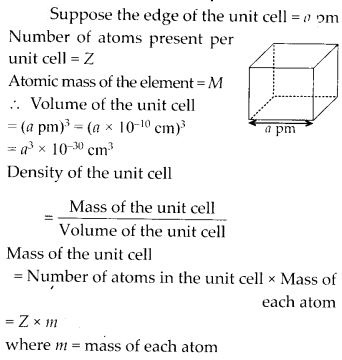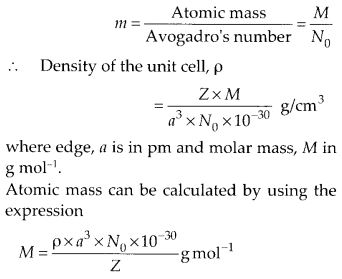Question 6.
‘Stability of a crystal is reflected in the magnitude of its melting point’. Comment. Collect melting points of solid water, ethyl alcohol, diethyl ether, and methane from a data book. What can you say about the intermolecular forces between these molecules?
Solution:
The melting points of some compounds are given below :
Water = 273 K, Ethyl alcohol = 155.7 K, Diethyl ether = 156.8 K, Methane = 90.5 K

The higher the melting point, the stronger are the forces holding the constituent particles together and hence greater is the stability.

The intermolecular forces in water and ethyl alcohol are mainly hydrogen bonding. The higher melting point of water as compared to alcohol shows that hydrogen bonding in ethyl alcohol molecules is not as strong as in water molecules. Diethyl ether is a polar molecule. The intermolecular forces present in them are the dipole-dipole attraction. Methane is a non-polar molecule. The only forces present in them are the weak van der Waals forces (London dispersion forces).

Question 7.
How will you distinguish between the following pairs of terms?

1. Hexagonal close-packing and cubic close¬packing
2. Crystal lattice and unit cells
3. Tetrahedral void and octahedral void

Solution:
(i) Hexagonal close-packing (hep) : The first layer is formed utilizing maximum space, thus wasting minimum space. In every second row, the particles occupy the depressions (also called voids) between the particles of the row (fig.). In the third row, the particles are vertically aligned with those in the first row giving AB AB AB …….. arrangement. This structure has hexagonal symmetry and is known as a hexagonal close-packing (hep) structure. This packing is more efficient and leaves small space which is unoccupied by spheres. In two dimensions central sphere is in contact with six other spheres. Only 26% space is free. In three dimensions, the coordination number is 12. A single unit cell has 4 atoms.leaves third layer not resembling with either first or second layer, but the fourth layer is similar to first, the fifth layer to second, sixth to third
and so on giving pattern ABC ABC ABC ……. This arrangement has cubic symmetry and is known as a cubic close-packed (cep) arrangement. This is also called face-centered cubic (fee).

(ii) Crystal lattice: A regular arrangement of the constituent particles (i.e., atoms, ions, or molecules) of a crystal in three-dimensional space is called crystal lattice or space lattice.

Unit cells: The smallest three-dimensional portion of a complete space lattice which when repeated over and over again in different directions produces the complete space lattice is called the unit cell.

(iii) The empty spaces left between closed packed spheres are called voids or holes.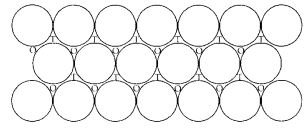(a) Octahedral voids: This void is surrounded by six spheres and formed by a combination of two triangular voids of the first and second layers. There is one octahedral void per atom in a crystal. The radius ratio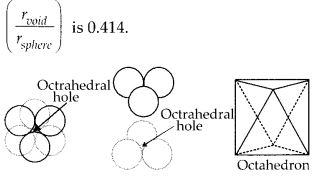(b) Tetrahedral voids: These voids are surrounded by four spheres that lie at the vertices of a regular tetrahedron. There are two tetrahedral voids per atom in a crystal and the radius ratio ($\frac { { r }_{ void } }{ { r }_{ sphere } }$) is 0.225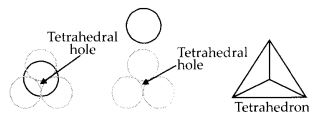Question 8.
How many lattice points are there in one unit cell of each of the following lattices?

1. Face–centered cubic
2. Face – centred tetragonal
3. Body – centered

Solution:
Lattice points in face-centred cubic or face-centred tetragonal = 8 (at corners) + 6 (at face-centres) = 14
Lattice points in body-centred cube = 8 (at corners) + 1 (at body-centre) = 9

Question 9.
Explain:

1. The basis of similarities and differences between metallic and ionic crystals.
2. Ionic solids are hard and brittle.

Solution:
(i) Similarities: Both ionic and metallic crystals have electrostatic forces of attraction. In ionic crystals, these are between oppositely charged ions. In metals, these are among the valence electrons and the kernels. That is why both have non-directional bonds.

Differences : (a) In ionic crystals, the ions are not free to move. Hence, they cannot conduct electricity in the solid-state. They can do so only in the molten state or in an aqueous solution. In metals, the valence electrons are free to move. Hence, they can conduct electricity in the solid-state.

(b) Ionic bond is strong due to electrostatic forces of attraction. Metallic bonds may be weak or strong depending upon the number of valence electrons and the size of the kernels.

(ii) Ionic crystals are hard because they have strong electrostatic forces of attraction among the oppositely charged ions. They are brittle because the ionic bond is non-directional.

Question 10.
Calculate the efficiency of packing in the case of a metal crystal for

1. simple cubic
2. face-centered cubic (with the assumption that atoms are touching each other).
3. body-centered cubic

Solution: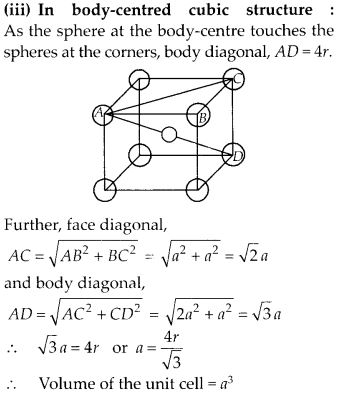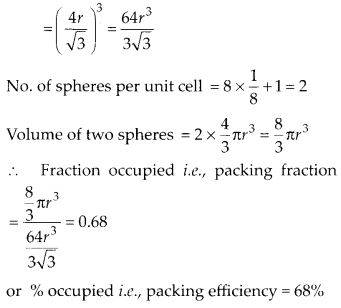Question 11.
Silver crystallizes in fee lattice. If the edge length of the cell is 4.07 × 10-8 cm and density is 10.5 cm-3, calculate the atomic mass of silver.
Solution:Question 12.
A cubic solid is made of two elements P and Q. Atoms of Q are at the corners of the cube and P at the body center. What is the formula of the compound? What are the coordination numbers of P and Q?
Solution:
As atoms Q are present at the eight corners of the cube, therefore the number of atoms of Q in the unit cell =$\frac { 1 }{ 8 }$ × 8 = 1
As atoms P are present at the body-center, therefore, the number of atoms of P in the unit cell = 1
Hence, the formula of the compound is PQ. The coordination number of each of P and Q = 8

Question 13.
Niobium crystallizes in a body-centered cubic structure. If the density is 8.55 g cm-3, calculate the atomic radius of niobium using its atomic mass 93 u.
Solution:
Given d = 8.55 g cm-3, M = 93 g mol-1, Z = 2 (for bcc), NA = 6.022 × 1023, r = ?
Using formula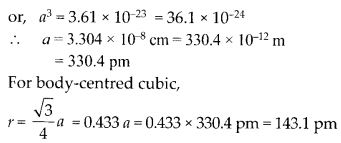Question 14.
If the radius of the octahedral void is r and the radius of the atoms in close-packing is R, derive a relation between r and R.
Solution:
R and r are the radii of the octahedral site and atoms respectively, then from Pythagoras theorem we get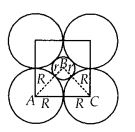AC2 = AB2 + BC2
(2 R)2 = (R + r)2 + (R + r)2
or,$\sqrt { 2 }$R = R + r
or, ($\sqrt { 2 }$-1) R = r
∴ r = 0.414 R

Question 15.
Copper crystallizes into a fee lattice with an edge length of 3.61 × 10-8 cm. Show that the calculated density is in agreement with its measured value of 8.92 g cm-3.
Solution:Question 16.
Analysis shows that nickel oxide has the formula Ni0.98 O1.00. What fractions of nickel exist as Ni2+ and Ni3+ ions?
Solution:
98 Ni-atoms are associated with 100 O – atoms. Out of 98 Ni-atoms, suppose Ni present as Ni2+ = x
Then Ni present as Ni3+ = 98 – x
The total charge on x Ni2+ and (98 – x) Ni3+ should
be equal to charge on 100 O2- ions.
Hence, x × 2 + (98 – x) × 3 = 100 × 2 or 2x + 294 – 3x = 200 or x = 94
∴ Fraction of Ni present as Ni2+ =$\frac { 94 }{ 98 }$ × 100 = 96%
Fraction of Ni present as Ni3+ =$\frac { 4 }{ 98 }$ × 100 = 4%

Question 17.
What is a semiconductor? Describe the two main types of semiconductors and contrast their conduction mechanism.
Solution:
Those solids which have intermediate conductivities ranging from 10-6 to 104 ohm-1 m-1 are classified as semiconductors. As the temperature rises, there is a rise in conductivity value because electrons from the valence band jump to the conduction band.

(i) n-type semiconductor: When a silicon or germanium crystal is doped with group 15 elements like P or As, the dopant atom forms four covalent bonds like Si or Ge atom but the fifth electron, not used in bonding, becomes delocalized and continues its share towards electrical conduction. Thus silicon or germanium doped with P or As is called an H-type semiconductor, a-indicative of negative since it is the electron that conducts electricity.

(ii) p-type semiconductor: When silicon or germanium is doped with group 13 elements like B or Al, the dopant is present only with three valence electrons. An electron vacancy or a hole is created at the place of the missing fourth electron. Here, this hole moves throughout the crystal-like a positive charge giving rise to electrical conductivity. Thus Si or Ge doped with B or Al is called a p-type semiconductor, p stands for the positive hole since it is the positive hole that is responsible for conduction.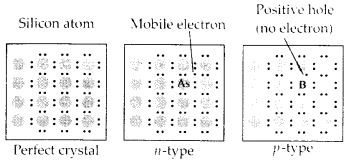Question 18.
Non-stoichiometric cuprous oxide, Cu2O can be prepared in the laboratory. In this oxide, the copper to oxygen ratio is slightly less than 2:1. Can you account for the fact that this substance is a p-type semiconductor?
Solution:
The ratio less than 2: 1 in Cu20 shows cuprous (Cu+) ions have been replaced by cupric (Cu2+) ions. For maintaining electrical neutrality, every two Cu+ ions will be replaced by one Cu2+ ion thereby creating a hole. As conduction will be due to the presence of these positive holes, hence it is a p-type semiconductor.

Question 19.
Ferric oxide crystallizes in a hexagonal dose-packed array of oxide ions with two out of every three octahedral holes occupied by ferric ions. Derive the formula of the ferric oxide.
Solution:
Suppose the number of oxide ions (O2-) in the packing = 90
∴ Number of octahedral voids = 90
As 2/3rd of the octahedral voids are occupied by ferric ions, therefore, number of ferric ions 2 present =$\frac { 2 }{ 3 }$ × 90 = 60
∴ Ratio of Fe3+ : O2- = 60 : 90 = 2 : 3
Hence, the formula of ferric oxide is Fe2O3.

Question 20.
Classify each of the following as being either a p-type or n-type semiconductor :

1. Ge doped with In
2. B doped with Si.

Solution:

1. Ge is group 14 element and In is group 13 element. Hence, an electron-deficient hole is created, and therefore, it is a p-type semiconductor.
2. Bis group 13 element and Si is group 14 element, there will be a free electron, So, it is an n-type semiconductor.

Question 21.
Gold (atomic radius = 0.144 nm) crystallizes in a face-centered unit cell. What is the length of a side of the cell?
Solution:
Given r = 0.144 nm, a = ?
For fcc, a = 2$\sqrt { 2 }$ r = 2 × 1.414 × 0.144 nm = 0.407 nm

Question 22.
In terms of band theory, what is the difference

1. between a conductor and an insulator
2. between a conductor and a semiconductor?

Solution:
In most of the solids and in many insulating solids conduction takes place due to the migration of electrons under the influence of an electric field. However, in ionic solids, it is the ions that are responsible for the conducting behavior due to their movement.

(i) In metals, conductivity strongly depends upon the number of valence electrons available in an atom. The atomic orbitals of metal atoms from molecular orbitals are so close in energy to each other, as to form a band. If this band is partially filled or it overlaps with the higher energy unoccupied conduction band, then electrons can flow easily under an applied electric field and the metal behaves as a conductor.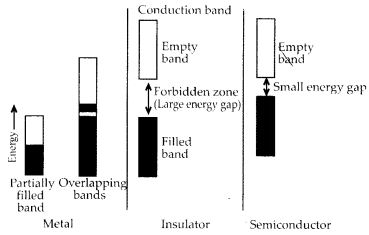If the gap between the valence band and the next higher unoccupied conduction band is large, electrons cannot jump into it and such a substance behaves as an insulator.

(ii) If the gap between the valence band and conduction band is small, some electrons may jump from the valence band to the conduction band. Such a substance shows some conductivity and behaves as a semiconductor. The electrical conductivity of semiconductors increases with an increase in temperature since more electrons can jump to the conduction band. Silicon and germanium show this type of behavior and are called intrinsic semiconductors. Conductors have no forbidden band.

Question 23.
Explain the following terms with suitable examples :

1. Schottky defect
2. Frenkel defect
3. Interstitial defect
4. F-centres.

Solution:
(i) Schottky defect: In Schottky defect, a pair of vacancies or holes exist in the crystal lattice due to the absence of an equal number of cations and anions from their lattice points. It is a common defect in ionic compounds of high coordination number where both cations and anions are of the same size, e.g., KCl, NaCl, KBr, etc. Due to this defect density of crystal decreases and it begins to conduct electricity to a smaller extent.

(ii) Frenkel defect: This defect arises when some of the ions in the lattice occupy interstitial sites leaving lattice sites vacant. This defect is generally found in ionic crystals where anion is much larger in size than the cation, e.g., AgBr, ZnS, etc. Due to this defect density does not change, electrical conductivity increases to a small extent and there is no change in overall chemical composition of the crystal.(iii) Interstitial defect: When some constituent particles (atoms or molecules) occupy an interstitial site of the crystal, it is said to have an interstitial defect. Due to this defect the density of the substance increases.

(iv) F-Centres: These are the anionic sites occupied by unpaired electrons. F-centres impart color to crystals. The color results from the excitation of electrons when they absorb energy from the visible light falling on the crystal.

Question 24.
Aluminum crystallizes in a cubic close-packed structure. Its metallic radius is 125 pm.

1. What is the length of the side of the unit cell?
2. How many unit cells are there in 1.00 cm3 of aluminum?

Solution:Question 25.
If NaCI is doped with 10-3 mol % SrCl2, what is the concentration of cation vacancies?
Solution:
Let moles of NaCI = 100
∴ Moles of SrCl2 doped = 10-3
Each Sr2+ will replace two Na+ ions. To maintain electrical neutrality it occupies one position and thus creates one cation vacancy.
∴ Moles of cation vacancy in 100 moles NaCI = 10-3
Moles of cation vacancy in one mole
NaCI = 10-3 × 10-2 = 10-5
∴ Number of cation vacancies
= 10-5 × 6.022 × 1023 = 6.022 × 1018 mol-1

Question 26.
Explain the following with suitable example:

1. Ferromagnetism
2. Paramagnetism
3. Ferrimagnetism
4. Antiferromagnetism
5. 12-16 and 13-15 group compounds.

Solution:
(i) Ferromagnetic substances: Substances that are attracted very strongly by a magnetic field are called ferromagnetic substances, e.g., Fe, Ni, Co, and CrO2 show ferromagnetism. Such substances remain permanently magnetized, once they have been magnetized. This type of magnetic moment is due to unpaired electrons in the same direction.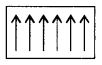The ferromagnetic material, CrO2, is used to make magnetic tapes used for audio recording.

(ii) Paramagnetic substances: Substances that are weakly attracted by the external magnetic field are called paramagnetic substances. The property thus exhibited is called paramagnetism. They are magnetized in the same direction as that of the applied field. This property is shown by those substances whose atoms, ions, or molecules contain unpaired electrons, e.g., O2, Cu2+, Fe3+, etc. These substances, however, lose their magnetism in the absence of a magnetic field.

(iii) Ferrimagnetic substances: Substances that are expected to possess large magnetism on the basis of the unpaired electrons but actually have small net magnetic moment are called ferrimagnetic substances, e.g., Fe3O4, ferrites of the formula M2+Fe2O4 where M = Mg, Cu, Zn, etc. Ferrimagnetism arises due to the unequal number of magnetic moments in opposite directions resulting in some net magnetic moment.(iv) Antiferromagnetic substances: Substances that are expected to possess paramagnetism or ferromagnetism on the basis of unpaired electrons but actually they possess zero net magnetic moments are called antiferromagnetic substances, e.g., MnO. Antiferromagnetism is due to the presence of an equal number of magnetic moments in the opposite directions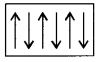(v) 13-15 group compounds: When the solid-state materials are produced by a combination of elements of groups 13 and 15, the compounds thus obtained are called 13-15 compounds. For example, InSb, AlP, GaAs, etc.

12-16 group compounds: Combination of elements of groups 12 and 16 yields some solid compounds which are referred to like 12-16 compounds. For example, ZnS, CdS, CdSe, HgTe, etc. In these compounds, the bonds have an ionic character.

#### Plus Two Chemistry Chapter Wise Textbook Solutions

HSE Kerala Plus Two syllabus, Previous year question papers, and other study materials.

### Plus Two Chemistry Related Links

We hope the given HSE Kerala Board Syllabus Plus Two ChemistryTextbook Solutions Chapter Wise Pdf Free Download in both English Medium and Malayalam Medium will help you.

If you have any queries regarding Higher Secondary Kerala Plus Two   NCERT syllabus, drop a comment below and we will get back to you at the earliest.

Keralanotes.com      Keralanotes.com      Keralanotes.com      Keralanotes.com      Keralanotes.com